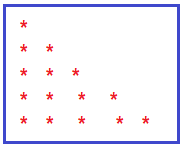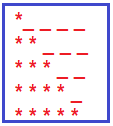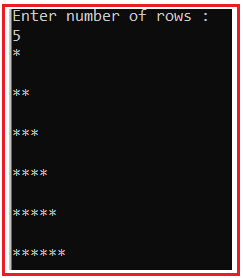# Right Triangle Star Pattern in C#

## Right Triangle Star Pattern Program in C# with Examples

In this article, I am going to discuss How to implement the Right Triangle Star Pattern Program in C# with Examples. Let us understand this with an example. Please have a look at the below image which shows the Right triangle star pattern.##### Understanding the Right Triangle Star Pattern
1. In the first row, we want one star and 4 blank spaces.
2. In the second row, we want 2 stars and 3 blank spaces.
3. In the third row, we want 3 stars and 2 blank spaces.
4. In the fourth row, we want 4 stars and 1 blank space.
5. In the fifth row, we want 5 stars and 0 blank spaces.
6. This list will go on as of the number of rows.1. From the above pattern, it is clear that at each row number of stars is increasing +1 at each row.
2. For example, the 1st row has 1 star, the 2nd row has 2 stars and the 3rd row has 3 stars, and so on.
3. From the above pattern, it is clear that at each row number of blank spaces are decreasing –1 at each row. For example, the 1st row has 4 spaces, the 2nd row has 3 spaces, the 3rd row has 2 spaces, and so on.
##### How to Implement the Right Triangle Star Pattern in C#?

To solve the above pattern,

1. We are going to use two for loops. The outer for loop and the inner for loop.
2. The outer for loop will be used to handle the rows one by one and the inner for loop will be used to handle the columns one by one.
3. From the above explanation, it is clear that the number of stars in each row is equal to the current row.
4. 1st row has 1 star, 2nd row has 2 stars, and so on.
5. The inner loop will be used to print the stars according to row and the outer loop job is to go on a new line after printing the current row stars.
##### C# Program to Print Right Angle Triangle Star Pattern

The following C# Program will allow the user to input the number of rows and then print the right angle triangle start pattern on the console.

```using System;
public class AreaOfRectangle
{
public static void Main()
{
Console.WriteLine("Enter number of rows :");

for (int i = 0; i <= rows; i++)
{
for (int j = 0; j <= i; j++)
{
Console.Write("*");
}
Console.WriteLine("\n");
}

}
}
```
###### Output:In the next article, I am going to discuss Mirrored Right Angle Triangle Star Pattern Program in C# with Examples. Here, in this article, I try to explain Right Triangle Star Pattern Program in C# with Examples and I hope you enjoy this Right Triangle Star Pattern Program in the C# article.

### 1 thought on “Right Triangle Star Pattern in C#”

1.the variables int ‘i’ and ‘j’ should be initialize with 1 instead of 0.
now it is printing 6 rows instead of 5 rows (as user requested).
Correct me if I’m wrong.